# 机器人学编程简介~2019~

13 篇文章 19 订阅

• 什么是机器人？

• 机器人的应用场合？

• 如何构建机器人？

• 如何编程机器人？

• 什么是最好的机器人编程语言？

• 如何使用JAVA编程机器人？

• 什么是机器人工程？

• 机器人过程自动化（RPA）和机器人编程有什么区别？

• 谁发明了世界上第一个机器人？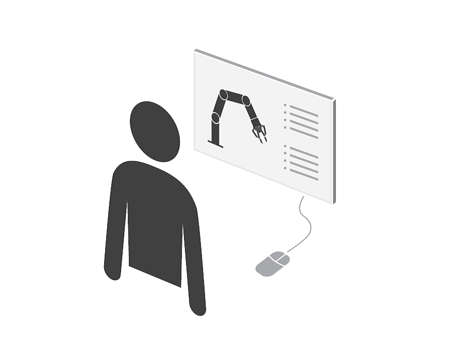1. C / C ++

2. Python

3. Java

4. C＃ / .NET
C＃是Microsoft提供的专有编程语言。我在这里包含C＃/ .NET主要是​​因为Microsoft Robotics Developer Studio使用它作为主要语言。如果您打算使用这个系统，您可能不得不使用C＃。但是，首先学习C / C ++可能是长期开发编码技能的好选择。

5. MATLAB
MATLAB及其开源亲属，如Octave，在一些机器人工程师中非常受欢迎，用于分析数据和开发控制系统。还有一个非常受欢迎的MATLAB 机器人工具箱。我认识那些仅使用MATLAB开发整个机器人系统的人。如果您想分析数据，生成高级图形或实现控制系统，您可能需要学习MATLAB。

### 机器人编程入门教程（翻译）

• 数学 - 我们将使用一些三角函数和向量
• Python-Python是最流行的基本机器人编程语言之一 - 我们将使用基本的Python库和函数

## 可编程机器人的挑战：感知与现实以及控制的脆弱性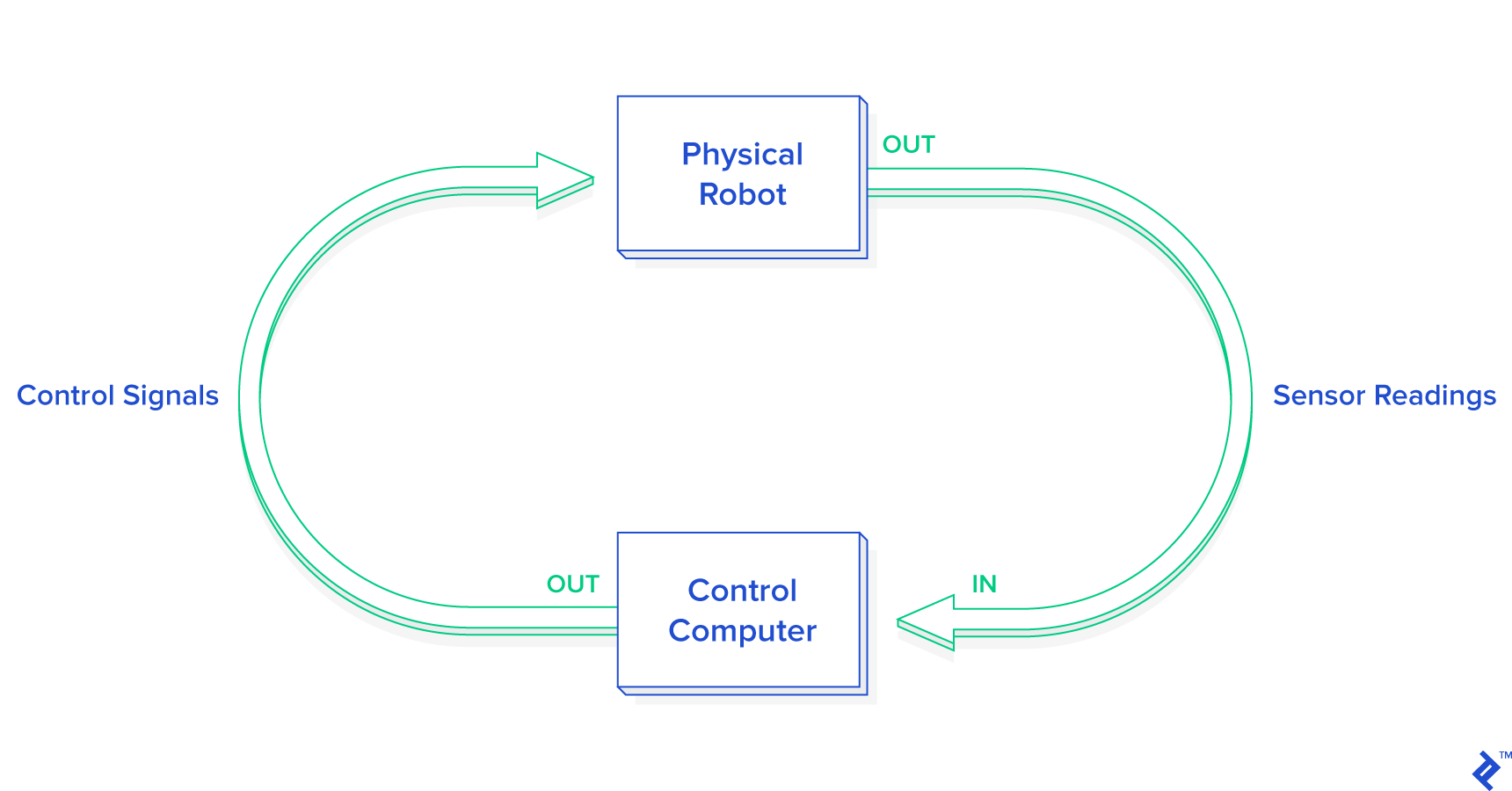[旁注：哲学家和心理学家都会注意到，生物也会依赖于他们自己对感官告诉他们的内在感知。机器人技术的许多进步来自于观察生物并观察它们对意外刺激的反应。想一想。你的世界内部模型是什么？它与蚂蚁和鱼的不同？（希望如此。）然而，就像蚂蚁和鱼一样，它可能会过度简化世界的某些现实。当你对世界的假设不正确时，它会让你面临失去控制权的风险。有时候我们称之为“危险”。就像我们的小机器人在未知宇宙中挣扎求生存一样，我们所有人也是如此。这是机器人专家的强大见解。]

## 可编程机器人仿真模拟器

• models/supervisor.py - 这个类负责机器人周围的模拟世界和机器人本身之间的相互作用。它演变了我们的机器人状态机并触发控制器以计算所需的行为。
• models/supervisor_state_machine.py- 该类表示机器人可以处于的不同状态，具体取决于其对传感器的解释。
• 目录中的models/controllers文件 - 这些类在给定已知环境状态的情况下实现机器人的不同行为。特别地，根据状态机选择特定控制器。

## 可编程机器人

### API

• read_proximity_sensors() 以传感器的本机格式返回九个值的数组
• read_wheel_encoders() 返回一个包含两个值的数组，表示自开始以来的总计滴答数
• set_wheel_drive_rates( v_l, v_r ) 取两个值（以弧度/秒为单位）并将轮子的左右速度设置为这两个值

## 仿真模拟器

• 将物理规则应用于机器人的运动
• 考虑与障碍物的碰撞
• 为机器人传感器提供新值

# step the simulation through one time interval
def step( self ):
dt = self.dt
# step all the robots
for robot in self.robots:
# step robot motion
robot.step_motion( dt )

# apply physics interactions
self.physics.apply_physics()

# NOTE: The supervisors must run last to ensure they are observing the "current" world
# step all of the supervisors
for supervisor in self.supervisors:
supervisor.step( dt )

# increment world time
self.world_time += dt


apply_physics()功能在内部更新机器人接近传感器的值，以便管理员能够在当前模拟步骤中估计环境。相同的概念适用于编码器。

## 一个简单的模型

• 地形总是平坦而均匀
• 障碍永远不会是圆的
• 车轮永不滑动
• 什么都不会推动机器人
• 传感器永远不会失败或给出错误的读数
• 轮子在被告知时总是转动

## 控制回路

1. 应用控制信号。
2. 测量结果。
3. 生成新的控制信号，使我们更接近目标。

## 一个漂亮的技巧：简化模型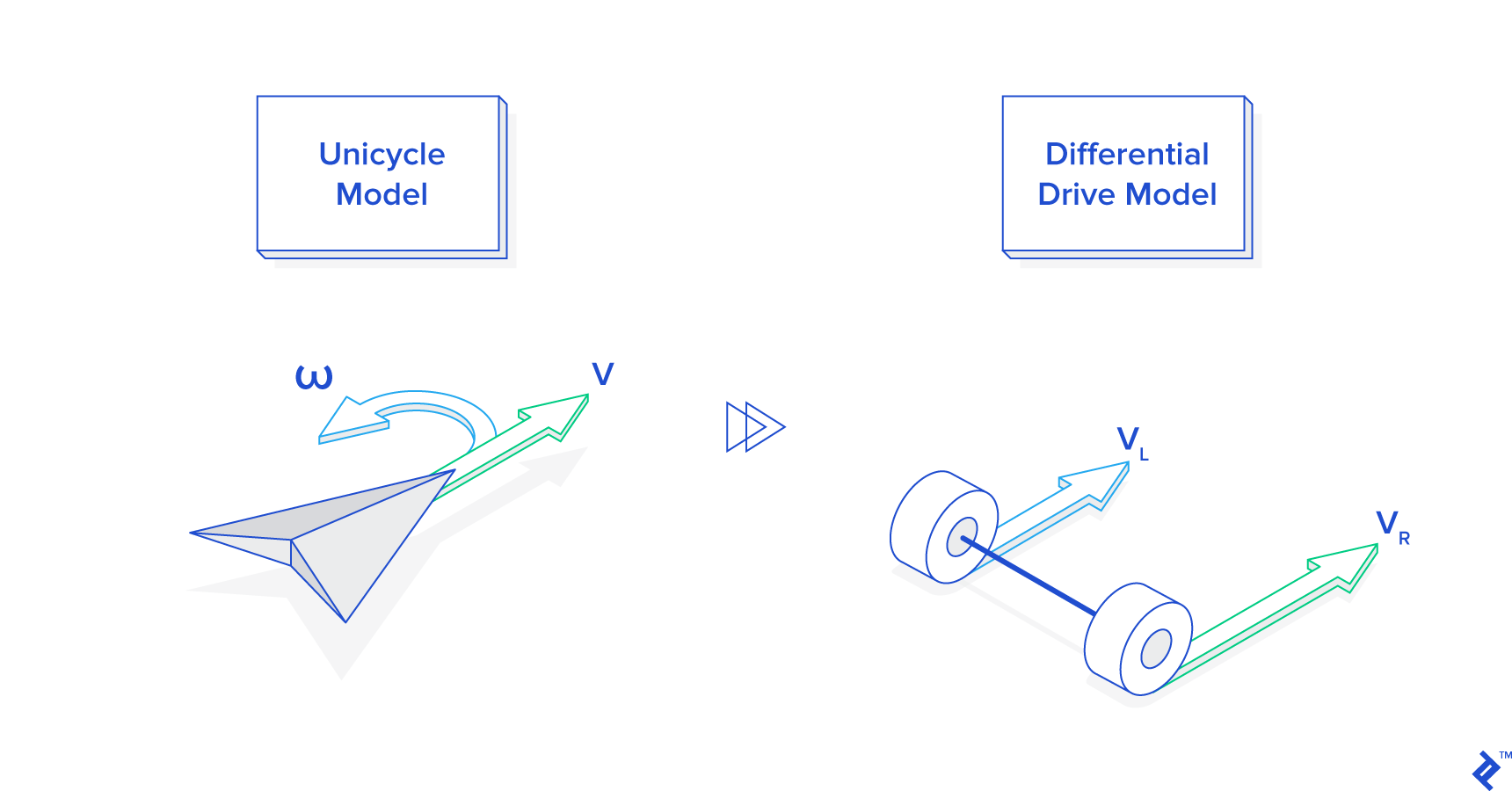# generate and send the correct commands to the robot
def _send_robot_commands( self ):
# ...
v_l, v_r = self._uni_to_diff( v, omega )
self.robot.set_wheel_drive_rates( v_l, v_r )

def _uni_to_diff( self, v, omega ):
# v = translational velocity (m/s)
# omega = angular velocity (rad/s)

L = self.robot_wheel_base_length

v_l = ( (2.0 * v) - (omega*L) ) / (2.0 * R)
v_r = ( (2.0 * v) + (omega*L) ) / (2.0 * R)

return v_l, v_r


## 估计状态：使机器人了解自己

• 障碍的方向
• 障碍物的距离
• 机器人的位置
• 机器人的标题

# update the distances indicated by the proximity sensors
def _update_proximity_sensor_distances( self ):
self.proximity_sensor_distances = [ 0.02-( log(readval/3960.0) )/30.0 for


# update the estimated position of the robot using it's wheel encoder readings
def _update_odometry( self ):
N = float( self.wheel_encoder_ticks_per_revolution )

# read the wheel encoder values

# get the difference in ticks since the last iteration
d_ticks_left = ticks_left - self.prev_ticks_left
d_ticks_right = ticks_right - self.prev_ticks_right

# estimate the wheel movements
d_left_wheel = 2*pi*R*( d_ticks_left / N )
d_right_wheel = 2*pi*R*( d_ticks_right / N )
d_center = 0.5 * ( d_left_wheel + d_right_wheel )

# calculate the new pose
prev_x, prev_y, prev_theta = self.estimated_pose.scalar_unpack()
new_x = prev_x + ( d_center * cos( prev_theta ) )
new_y = prev_y + ( d_center * sin( prev_theta ) )
new_theta = prev_theta + ( ( d_right_wheel - d_left_wheel ) / self.robot_wheel_base_length )

# update the pose estimate with the new values
self.estimated_pose.scalar_update( new_x, new_y, new_theta )

# save the current tick count for the next iteration
self.prev_ticks_left = ticks_left
self.prev_ticks_right = ticks_right


## Python机器人编程方法：前进目标行为

# return a go-to-goal heading vector in the robot's reference frame
# get the inverse of the robot's pose
robot_inv_pos, robot_inv_theta = self.supervisor.estimated_pose().inverse().vector_unpack()

# calculate the goal vector in the robot's reference frame
goal = self.supervisor.goal()
goal = linalg.rotate_and_translate_vector( goal, robot_inv_theta, robot_inv_pos )

return goal


# calculate the error terms

# calculate angular velocity
omega = self.kP * theta_d


self.kP在上面的控制器片段中，Python实现是一个控制增益。这是决定了我们如何快速转了系数比例如何远离我们所面对的目标。如果我们的标题中的错误是0，那么转弯率也是0。在文件中的真实Python函数中go_to_goal_controller.py，您将看到更多类似的增益，因为我们使用PID控制器而不是简单的比例系数。

# calculate translational velocity
# velocity is v_max when omega is 0,
# drops rapidly to zero as |omega| rises
v = self.supervisor.v_max() / ( abs( omega ) + 1 )**0.5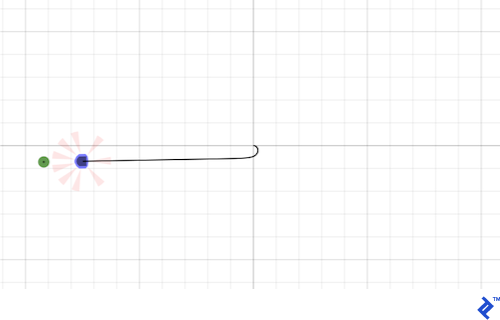## Python机器人编程方法：避免 - 障碍行为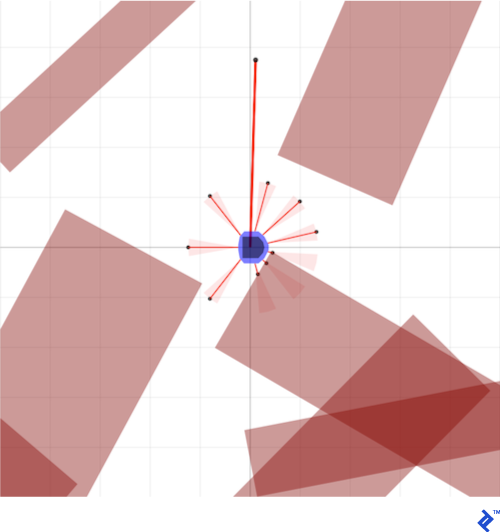# sensor gains (weights)
self.sensor_gains = [ 1.0+( (0.4*abs(p.theta)) / pi )
for p in supervisor.proximity_sensor_placements() ]

# ...

# return an obstacle avoidance vector in the robot's reference frame
# also returns vectors to detected obstacles in the robot's reference frame
# initialize vector
obstacle_vectors = [ [ 0.0, 0.0 ] ] * len( self.proximity_sensor_placements )
ao_heading_vector = [ 0.0, 0.0 ]

# get the distances indicated by the robot's sensor readings
sensor_distances = self.supervisor.proximity_sensor_distances()

# calculate the position of detected obstacles and find an avoidance vector
robot_pos, robot_theta = self.supervisor.estimated_pose().vector_unpack()

for i in range( len( sensor_distances ) ):
# calculate the position of the obstacle
sensor_pos, sensor_theta = self.proximity_sensor_placements[i].vector_unpack()
vector = [ sensor_distances[i], 0.0 ]
vector = linalg.rotate_and_translate_vector( vector, sensor_theta, sensor_pos )
obstacle_vectors[i] = vector   # store the obstacle vectors in the robot's reference frame

# accumulate the heading vector within the robot's reference frame
linalg.scale( vector, self.sensor_gains[i] ) )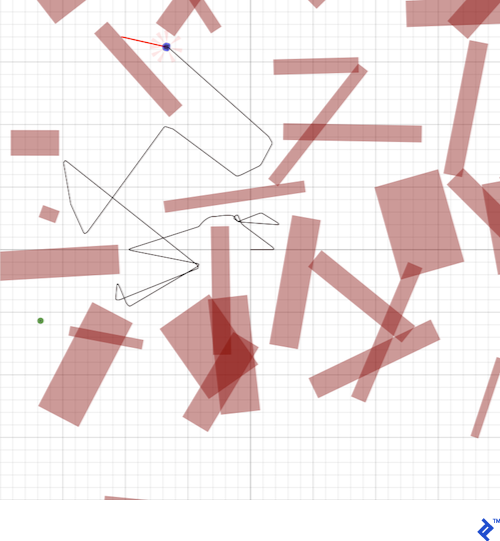## Python机器人编程方法：跟随墙行为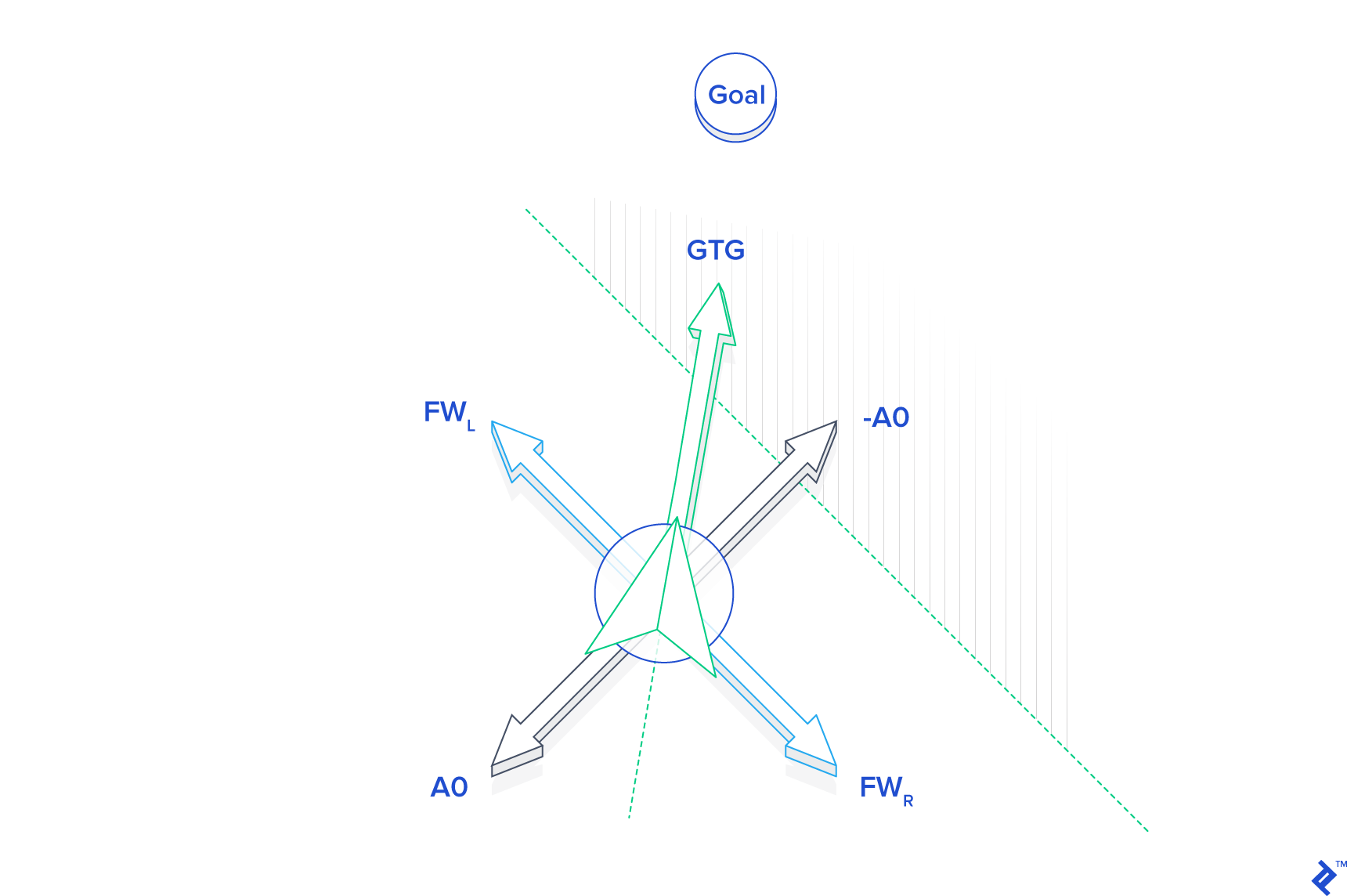## 最终控制设计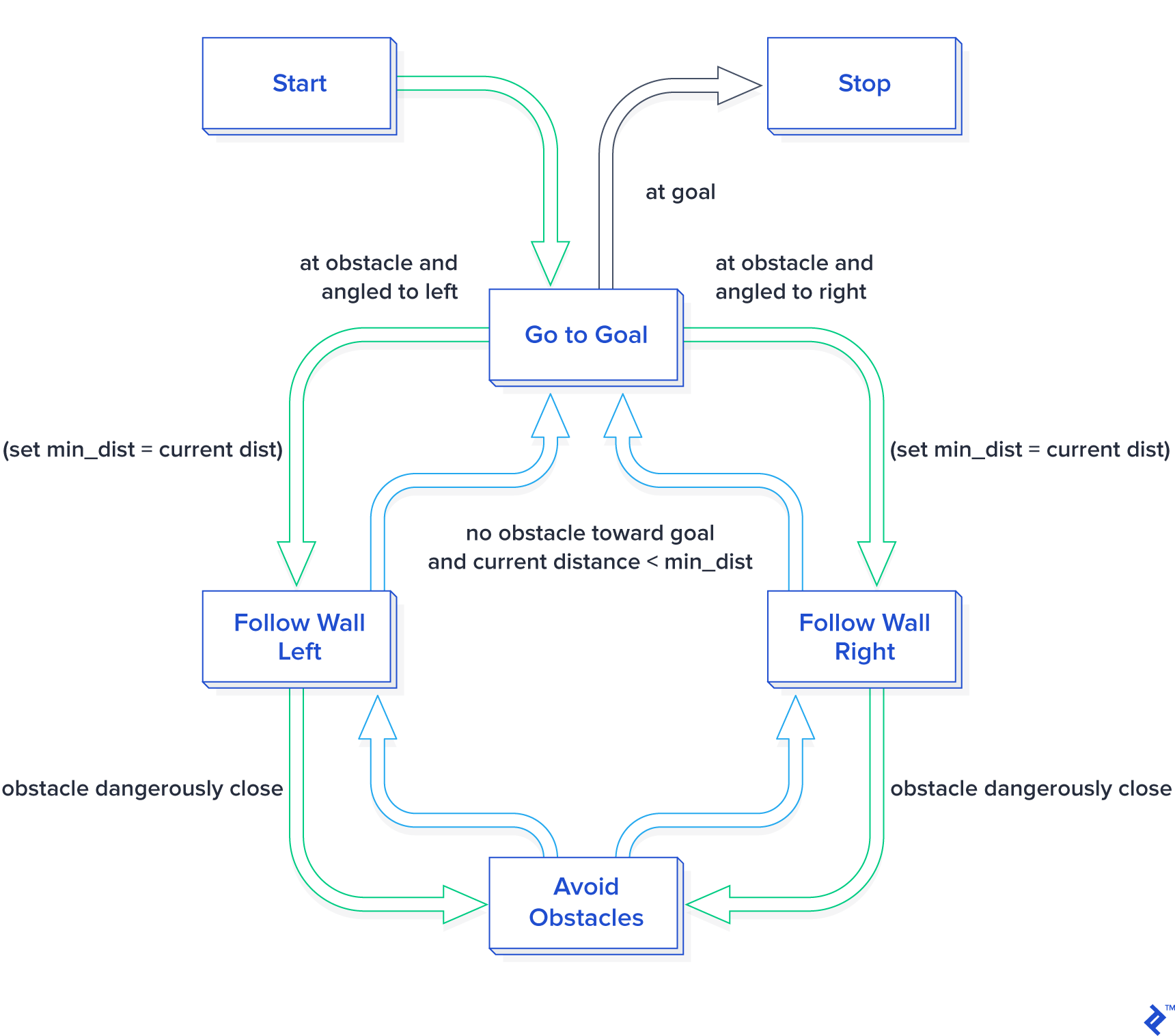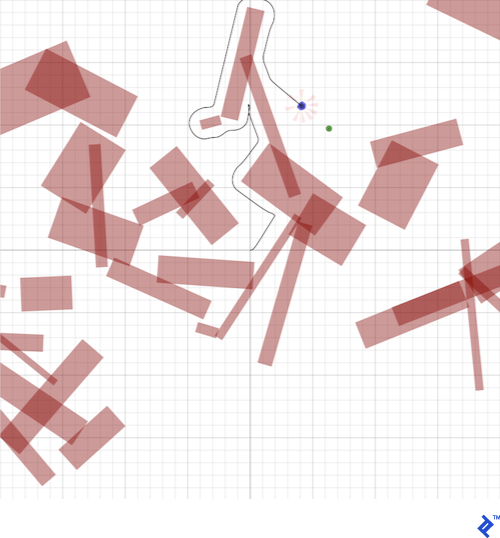## 调整，调整，调整：试用和错误

Sobot Rimulator附带的控制方案经过精心调校。这里需要花费很多时间来调整一个小变量，并在那里调整另一个等式，以使其以我满意的方式工作。机器人编程通常涉及大量简单的反复试验。机器人非常复杂，并且很少有捷径可以让它们在机器人模拟器环境中表现得最佳......至少，没有太多的直接机器学习，但这是另外一种蠕虫。

• kP每个控制器的错误增益
• 避免障碍物控制器使用的传感器增益
• 在每个控制器中计算v作为ω的函数
• 跟随控制器使用的障碍物间隔距离
• 使用的切换条件 supervisor_state_machine.py
• 几乎任何其他东西

## 什么来的样本

05-0213406-05218
12-19
03-05
08-051271
11-284万+
04-058万+
11-271509
06-135644
09-09103
07-2172
12-181425
01-294771
01-0289
07-042万+
12-273608
09-103182
03-09626
02-22219
©️2021 CSDN 皮肤主题: Age of Ai 设计师:meimeiellie点击重新获取扫码支付1.余额是钱包充值的虚拟货币，按照1:1的比例进行支付金额的抵扣。
2.余额无法直接购买下载，可以购买VIP、C币套餐、付费专栏及课程。余额充值